# What Is Velocity Used For? (10 Real Life Uses Of Velocity)

Velocity is not just a theoretical idea – it is a very a useful concept when applied to many fields, from physics to weather (and much more!)  Having a good grasp of velocity and how it is used will be helpful in many disciplines.

So, what are some uses of velocity?  Some uses of velocity include: firearms, flight, graphics, initial value problems, space travel, trajectories, travel, vehicle safety, water flow, and weather. Together with acceleration, velocity can help us to make predictions about where object will end up – and when.

Of course, in addition to telling you about how fast something is moving (speed), it can tell you the direction and where it will end up.

In this article, we’ll talk about 10 uses of velocity.  We’ll also get into the calculations for some examples that use velocity.

Let’s get started.

Hey! If you need to learn more about velocity and other math concepts for physics, check out this course:

Advanced Math For Physics: A Complete Self-Study Course

## What Is Velocity Used For? (10 Real Life Uses Of Velocity)

Velocity is used in lots of different ways in many fields.  Here are some specific examples of how we can use velocity in various disciplines:

• Firearms
• Flight
• Graphics
• Initial Value Problems
• Space Travel
• Trajectories
• Travel
• Vehicle Safety
• Water Flow
• Weather

Let’s take a closer look at how velocity is used in each of these fields, starting with firearms.

### Firearms

Velocity is used to make calculations related to firearms.  The muzzle velocity tells us how fast a bullet is moving when it comes out of the barrel of a gun.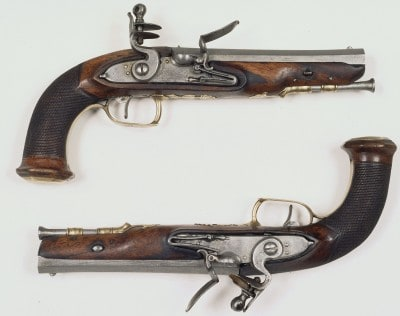The muzzle velocity of a firearm can tell us how far a bullet will travel in a given time (or before it hits the ground).

Muzzle velocity is often given in units of feet per second.  For example, a gun might have a muzzle velocity of 1,000 feet per second.

We can use the muzzle velocity to find out how long it will take a bullet to travel to a target.  With a muzzle velocity of 1,000 feet per second, it will take 0.3 seconds (300 milliseconds) to reach a target that is 300 feet away.

We can also use muzzle velocity to find out how far a bullet will travel until it hits the ground.  First, we need to find out how long it takes the bullet to fall to the ground.

This calculation is an initial value problem, using the height of the bullet (height of the gun barrel – let’s say 5 feet above ground) and gravity (32 feet per second, per second).  The acceleration (due to gravity) of the bullet towards the ground is -32, and antiderivatives tell us that the velocity would be -32t, and the height would be 5 – 16t2.

Solving for a height of zero (ground level), we get 0 = 5 – 16t2, or t = √(5/16), which is about 0.559 seconds.  With a muzzle velocity of 1,000 feet per second, the bullet would hit the ground at 1,000*0.559 = 559 feet away from the gun.

### Flight

Velocity is helpful in flight for several reasons.  First of all, we can calculate the time it will take to cover a given distance.

If the velocity V is constant, then the time T to cover a distance D is given by:

• T = D / V (time is equal to distance divided by velocity)

We should take the absolute value of the time we calculate to get a positive value (velocity can have a negative component, but time should be positive).

We can also use current velocity and acceleration (the derivative of velocity, and the second derivative of position) to predict our velocity at a future time.  The formula is given by:

• VF = VC + AT  (future velocity is equal to current velocity plus acceleration multiplied by time)

This assumes a constant acceleration.  If we do not have constant acceleration, we would need to integrate the acceleration over a time period.

We can also use the velocity (speed and direction) of the wind and the aircraft to make course corrections to stay on the correct heading.  As the wind changes, the aircraft’s velocity must also change.

### Graphics

Velocity is also used in graphics to create realistic animations.  Velocity tells how fast an object should move across the screen (pixel by pixel).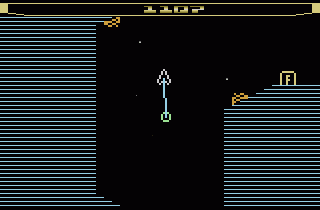Velocity can help us to figure out where and when objects in a video game or animated simulation will collide.

It can also tell us if two objects are going to collide on screen.  This is useful in flight simulations and other training programs.

### Initial Value Problems

Initial value problems in physics often use velocity to solve for the position or height of a moving object.  Sometimes, acceleration is also used in these problems.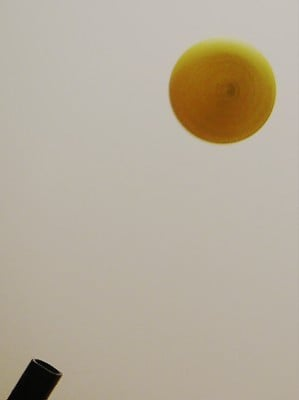We can use velocity in an initial value problem to find the height of a falling object at a specific time.

For example, given the velocity of an object, we would take the antiderivative to find the position function.  However, the function would have an unknown constant.

To find the value of the constant, we would use an initial value (the position of the object at a given time) in the position function.

If the velocity were a constant 60 miles per hour and the position were 200 at time t = 2, then we would have:

• V(t) = 60  [velocity function]
• P’(t) = 60  [velocity is the derivative of the position function]
• ∫P’(t)dt = ∫60dt  [integrate or take the antiderivative of both sides]
• P(t) = 60t + K  [the constant K in the position function is unknown]
• P(2) = 60(2) + K  [substitute a time of t = 2]
• 200 = 120 + K  [since the position is 200 at time t = 2]
• 80 = K

So, the position function is P(t) = 60t + 80.  We can use this function to find the position of the object at any time t.

### Space Travel

Velocity is used to calculate the velocity of objects in space, based on propulsion and momentum.  Remember that momentum is the product of mass and velocity:

• p = mv  [an object’s momentum equals mass times velocity]

For two objects in a collision, their momentum is equal:

• p1 = p2  [momentum of two objects is equal]
• m1v1 = m2v2  [use momentum equation for each object]

If a spacecraft at rest with a mass of 200,000 pounds uses a thruster that sends out 400 pounds of mass at a speed of 200 miles per hour, then we can find the resulting velocity of the spacecraft:

• m1v1 = m2v2
• (200,000)*v1 = (200)*(400)
• 200,000v1 = 80,000
• v1 = 2.5 miles per hour

So, the spacecraft would be moving at 2.5 miles per hour, in a direction opposite that of the ejected mass.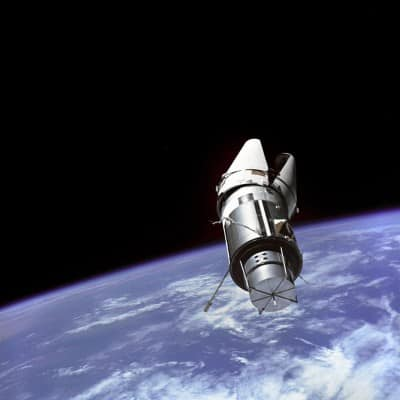Velocity is used in momentum calculations to figure out how fast a spacecraft will be moving after using a thruster.

### Trajectories

Velocity is also useful in calculating the trajectories of missiles and other ballistic objects.  Normally, the velocity of an object is broken down into component vectors, giving us velocity in two or three dimensions.

For example, consider a missile with a velocity of 100 miles per hour fired at an angle of 30 degrees from the horizontal (ground):

• The horizontal velocity (x-component) is 100*cos(30) = 100*(√3/2), or approximately 86.60 miles per hour.
• The vertical velocity (y-component) is 100*sin(30) = 100*(1/2), or 50 miles per hour.

Using the vertical velocity, we can solve an initial value problem (using gravity, wind resistance, and other factors) to find out when the missile will hit the ground (in terms of time).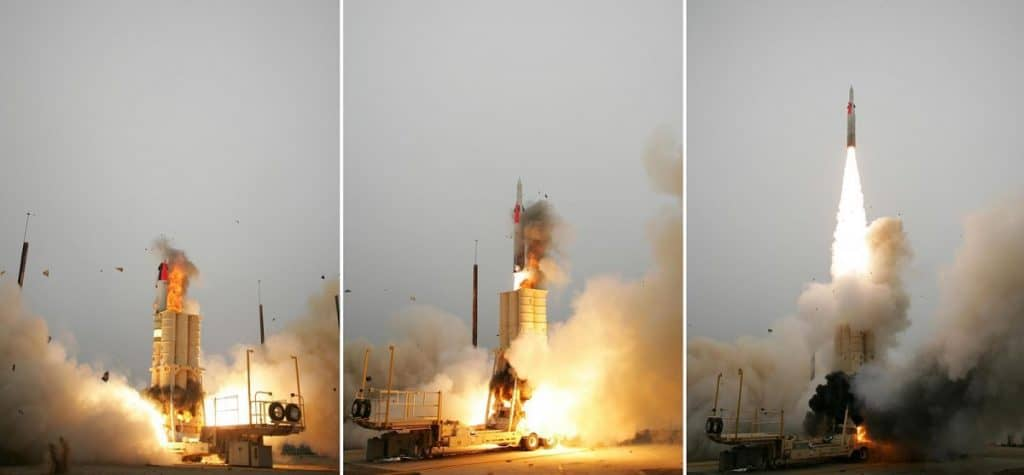We can use velocity as part of an initial value problem to find out where and where a missile will hit the ground.

We can then use the horizontal velocity and the time to find out how far the missile will travel before it hits the ground.  This will tell us where the missile will hit the ground.

### Travel

Velocity is used in travel by car, boat, or plane.  The key formula is given by:

• D = VT (Distance equals velocity times speed)

We use the absolute value of time to get a positive value (or use speed, which is the absolute value of velocity), since time and distance must be positive.

For example, if a car is traveling at 50 miles per hour, we can find out how long it will take to cover a distance of 375 miles:

• D = VT
• 375 = 50T
• 375/50 = T
• 7.5 = T

So, it will take 7.5 hours for the car to travel a distance of 50 miles.Velocity allows us to calculate how long it will take a vehicle to make a trip of a given distance.

A boat would need to use extra fuel to travel at the same speed against a strong tide (as opposed to moving in calm waters or moving with the tide).

### Vehicle Safety

Velocity is used in several ways in vehicle safety.  First, velocity is used to assess crash test safety ratings (how much damage is done to a vehicle and its occupants in collisions from various sides at a given velocity).

Velocity is also used to determine safe stopping distance for a vehicle in dry, rainy, and snowy/icy road conditions (stopping distance increases with higher velocity, less tire tread, and more slippery road conditions).

Velocity is also a part of what tells an airbag to deploy (along with other crash sensors in your vehicle).  A sudden change in velocity is one indicator that a vehicle has collided with another object.

### Water Flow

Velocity tells us how fast water flows through a pipe or hose.  Based on the size of the pipe (diameter), we can find out the flow rate (volume of water per unit of time).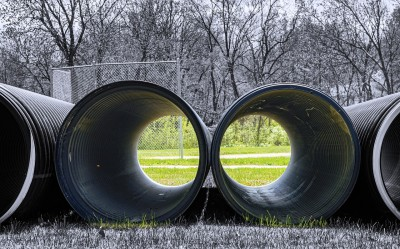Water velocity (along with pipe or hose diameter) can help us to determine the flow rate (volume per time unit).

For example, if the water in a pipe is flowing at 5 feet per second and the diameter of the pipe is 4 inches, we can find the flow rate as follows:

• Water Velocity = (5 feet per second)*(12 inches per foot) = 60 inches per second
• Cross-sectional area of pipe = area of circle with radius 2 inches = πR2 = π22 = 4π square inches
• Flow rate = (Water Velocity)*(Cross sectional area of pipe) = (60 inches per second)*(4π square inches) = 240π cubic inches per second, or about 754 cubic inches per second.

When we know the flow rate, we can figure out how long it will take to fill up a container of a given volume.

A container with a volume of 45,000 cubic inches will take 45,000 / 754 = 59.68 seconds (about 1 minute) to fill up with this pipe.

### Weather

Velocity has applications in weather reporting and prediction as well.  A high wind velocity makes it dangerous weather for boats and ships.

The velocity of wind also makes clouds and storms move faster.  Wind velocity also helps us to classify storms (hurricanes).

The wind velocity also adds to the wind chill factor, making a cold day seem even colder to us.

## Conclusion

Now you know how you can use velocity in various disciplines.  You also have a better understanding of the calculations involved in some of the applications of velocity.

You can learn about various uses of algebra in real life here.